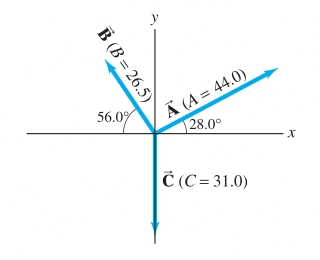# Problem: For the vectors shown in the figure, determinePart A. the magnitude of B − 3A. Express your answer using three significant figures.Part B. the direction of B −3A. Express your answer using three significant figures.

###### FREE Expert Solution

2D vectors, Magnitude & Direction/Components:

$\overline{)\mathbf{|}\stackrel{\mathbf{⇀}}{\mathbit{A}}\mathbf{|}{\mathbf{=}}\sqrt{{{\mathbit{A}}_{\mathbit{x}}}^{\mathbf{2}}\mathbf{+}{{\mathbit{A}}_{\mathbit{y}}}^{\mathbf{2}}}}$

$\overline{){\mathbf{tan}}{\mathbit{\theta }}{\mathbf{=}}\frac{{\mathbit{A}}_{\mathbit{y}}}{{\mathbit{A}}_{\mathbit{x}}}}$

Part A.

θA = 28.0°

A = Acosθ i - Asinθ j = 44.0cos28.0 i + 44.0sin28.0 j = 38.8 i + 20.7 j

94% (296 ratings)###### Problem Details

For the vectors shown in the figure, determinePart A. the magnitude of B − 3A. Express your answer using three significant figures.

Part B. the direction of −3A. Express your answer using three significant figures.